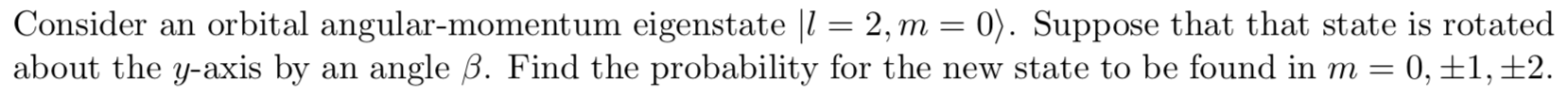# Consider an orbit al angular-momentum eigenstate l = 2, m = 0). Suppose that that state is rotated about the y-axis by an angle B. Find the probability for the new state to be found in m = 0,±1,±2

Question

It's a quantum mechanics question.help_outlineImage TranscriptioncloseConsider an orbit al angular-momentum eigenstate l = 2, m = 0). Suppose that that state is rotated about the y-axis by an angle B. Find the probability for the new state to be found in m = 0,±1,±2 fullscreen